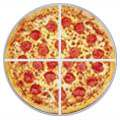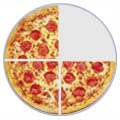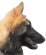# Improper Fractions74 (seven-fourths or seven-quarters)

An Improper Fraction has a top number larger than (or equal to) the bottom number.

It is usually "top-heavy"

## More Examples

 32 73 1615 1515 1005

See how the top number is bigger than (or equal to) the bottom number?
That makes it an Improper Fraction, (but there is nothing wrong about Improper Fractions).

## Three Types of Fractions

There are three types of fraction:## Fractions

A Fraction (such as 7/4) has two numbers:

NumeratorDenominator

The top number (the Numerator) is the number of parts we have.
The bottom number (the Denominator) is the number of parts the whole is divided into.

Example: 7/4 means:

• We have 7 parts
• Each part is a quarter (1/4) of a whole

So we can define the three types of fractions like this:

Proper Fractions: The numerator is less than the denominator Examples: 1/3, 3/4, 2/7 The numerator is greater than (or equal to) the denominator Examples: 4/3, 11/4, 7/7 A whole number and proper fraction together Examples: 1 1/3, 2 1/4, 16 2/5

## Improper Fraction

So an improper fraction is a fraction where the top number (numerator) is greater than or equal to the bottom number (denominator): it is top-heavy.44

## Can be Equal

What about when the numerator is equal to the denominator? For example 4/4 ?

Well it is the same as a whole, but it is written as a fraction, so most people agree it is a type of improper fraction.

## Improper Fractions or Mixed Fractions

We can use either an improper fraction or a mixed fraction to show the same amount.

For example 134 = 74, as shown here:

 134 74=## Converting Improper Fractions to Mixed Fractions

To convert an improper fraction to a mixed fraction, follow these steps:Divide the numerator by the denominator. Write down the whole number answer Then write down any remainder above the denominator.

### Example: Convert 114 to a mixed fraction.

Divide:

11 ÷ 4 = 2 with a remainder of 3

Write down the 2 and then write down the remainder (3) above the denominator (4).

2 34

That example can be written like this:### Example: Convert 103 to a mixed fraction.3 13

## Converting Mixed Fractions to Improper Fractions

To convert a mixed fraction to an improper fraction, follow these steps:Multiply the whole number part by the fraction's denominator. Add that to the numerator Then write the result on top of the denominator.

### Example: Convert 325 to an improper fraction.

Multiply the whole number part by the denominator:

3 × 5 = 15

15 + 2 = 17

Then write that result above the denominator:

175

We can do the numerator in one go:### Example: Convert 219 to an improper fraction.## Are Improper Fractions Bad ?

For mathematics they are actually better than mixed fractions. Because mixed fractions can be confusing when we write them in a formula: should the two parts be added or multiplied?

 Mixed Fraction: Improper Fraction: What is: 1 + 214   ? Is it: 1 + 2 + 14 = 314 ? Or is it: 1 + 2 × 14 = 112 ? What is: 1 + 94   ? It is: 44 + 94 = 134But, for everyday use, people understand mixed fractions better.

Example: It is easier to say "I ate 214 sausages", than "I ate 94 sausages"

We Recommend:

• For Mathematics: Improper Fractions
• For Everyday Use: Mixed Fractions